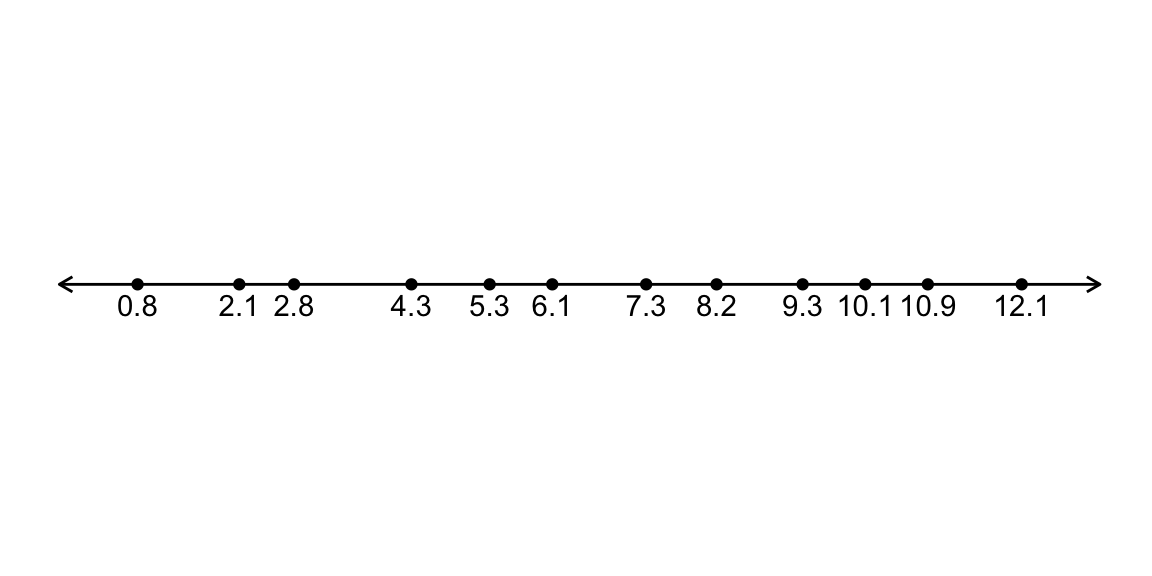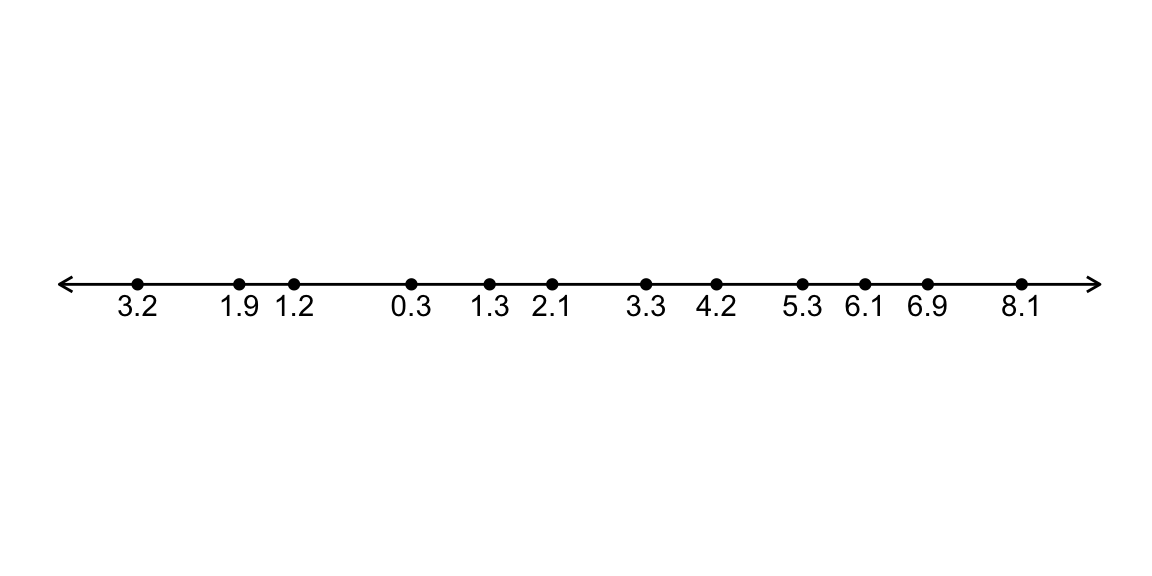# Wilcoxon Signed Rank Test

## Usual setting

Population: $$Y \sim$$ some population distribution

Sample: $$n$$ i.i.d from population: $$Y_1, \ldots, Y_n$$

Parameter: ?

Null Hypothesis the population ‘center’ is $$c_0$$.

Let’s talk about the procedure first, then come back to why it’s hard to be specific here.

## Wilcoxon Signed Rank Test Procedure

1. Find the distance of each observed value from the hypothesized center, $$c_0$$.
2. Assign a rank to each observation based on its distance from $$c_0$$: from 1 for closest, to $$n$$ for furthest from $$c_0$$.
3. Test statistic: $$S =$$ Sum of the ranks for the values that were larger than $$c_0$$.

## Example: test statistic calculation

$$H_0: c = 4$$1. Find distance to $$c_0 = 4$$.1. Assign ranks

2. Test statistic: $$S =$$ sum of ranks for $$Y_i > 4 =$$

## Reference distribution

Either:

• Use an exact p-value, by assuming each rank has the same chance of being assigned above or below $$c_0$$, or
• Use the Normal approximation to the null distribution of $$S$$

## Reference distribution: Exact p-values

If the population distribution were symmetric about $$c_0$$, each rank $$1,\ldots, n$$ independently has probability 0.5 of being assigned to an observation above $$c_0$$.

We can consider all possible ways of assigning the ranks $$1,\ldots, n$$ above and below $$c_0$$ to work out the exact reference distribution (this is what the R function wilcox.test() does if you use the argument exact = TRUE)

## Reference distribution: Normal approximation p-values

If the population distribution were symmetric about $$c_0$$,

$E(S) = \frac{n(n+1)}{4}, \quad Var(S) = \frac{n(n+1)(2n+1)}{24}$

(Can prove by considering $$S$$ as a sum of products between Bernoulli(0.5) r.v’s and the integers $$1, \ldots, n$$)

So, we can construct a Z-statistic

$Z = \frac{S - \frac{n(n+1)}{4}}{\sqrt{\frac{n(n+1)(2n+1)}{24}}}$

and compare it to a N(0, 1)

## Example: continued

$E(S) = \frac{n(n+1)}{4} = \frac{12(13)}{4} = 39$ $Var(S) = \frac{n(n+1)(2n+1)}{24} = \frac{12(13)(25)}{24} = 162.5$

$Z = \frac{66 - 39}{\sqrt{162.5}} = 2.12$

2 * (1 - pnorm(abs(z)))
##  0.03417047

## Performance of the Wilcoxon Signed Rank Test

As a test of the population mean:

• The Wilcoxon Signed Rank test is not assymptotically exact
• The Wilcoxon Signed Rank test is not consistent

As a test of the population median:

• The Wilcoxon Signed Rank test is not assymptotically exact
• The Wilcoxon Signed Rank test is not consistent

## Performance of the Wilcoxon Signed Rank Test

If you add an assumption: the population distribution is symmetric.

The Wilcoxon Signed Rank test is assymptotically exact

The Wilcoxon Signed Rank test is consistent

Null hypothesis: $$\mu = M = c_0$$

## Performance of the Wilcoxon Signed Rank Test

Often presented as:

“The nonparametric Wilcoxon signed rank test compares the median of a single column of numbers against a hypothetical median.” Incorrect, without symmetry assumption, and then it’s equally a test of the mean.

“This is another test that is a non-parametric equivalent of a 1-Sample t-test”. Incorrect, without symmetry assumption.

“The Wilcoxon signed-rank test applies to the case of symmetric continuous distributions. Under this assumption, the mean equals the median. The null hypothesis is $$H_0: \mu = \mu_0$$ Correct

## In R

y <- c(0.8, 2.1, 2.8, 4.3, 5.3, 6.1, 7.3, 8.2,
9.3, 10.1, 10.9, 12.1)

Exact p-values with exact = TRUE (default)

wilcox.test(y, mu = 4, exact = TRUE)
##
##  Wilcoxon signed rank test
##
## data:  y
## V = 66, p-value = 0.03418
## alternative hypothesis: true location is not equal to 4

## In R

y <- c(0.8, 2.1, 2.8, 4.3, 5.3, 6.1, 7.3, 8.2,
9.3, 10.1, 10.9, 12.1)

Approximate p-values with exact = FALSE and no continuity correction

wilcox.test(y, mu = 4, exact = FALSE, correct = FALSE)
##
##  Wilcoxon signed rank test
##
## data:  y
## V = 66, p-value = 0.03417
## alternative hypothesis: true location is not equal to 4